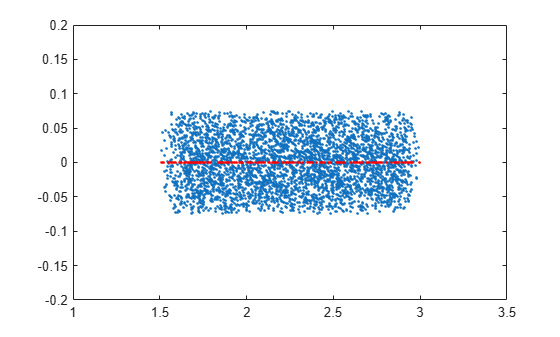Documentation

# complexify

Replace `ureal` atoms by summations of `ureal` and `ucomplex` (or `ultidyn`) atoms

## Syntax

```MC = complexify(M,alpha)
MC = complexify(M,alpha,'ultidyn')
```

## Description

The command `complexify` replaces `ureal` atoms with sums of `ureal` and `ucomplex` atoms using `usubs`. Optionally, the sum can consist of a `ureal` and `ultidyn` atom.

`complexify` is used to improve the conditioning of robust stability calculations (`robstab`) for situations when there are predominantly `ureal` uncertain elements.

`MC = complexify(M,alpha)` results in each `ureal` atom in `MC` having the same `Name` and `NominalValue` as the corresponding `ureal` atom in M. If `Range` is the range of one `ureal` atom from `M`, then the range of the corresponding ureal atom in `MC` is

[`Range(1)+alpha*diff(Range)/2 Range(2)-alpha*diff(Range)/2`]

The net effect is that the same real range is covered with a real and complex uncertainty. The real parameter range is reduced by equal amounts at each end, and `alpha` represents (in a relative sense) the reduction in the total range. The `ucomplex` atom will add this reduction in range back into `MC`, but as a ball with real and imaginary parts.

The `ucomplex` atom has `NominalValue` of 0, and `Radius` equal to `alpha*diff(Range)`. Its name is the name of the original `ureal` atom, appended with the characters `'_cmpxfy'`.

`MC = complexify(M,alpha,'ultidyn')` is the same, except that gain-bounded `ultidyn` atoms are used instead of `ucomplex` atoms. The `ultidyn` atom has its `Bound` equal to `alpha*diff(Range)`.

## Examples

collapse all

To illustrate complexification, create a uncertain real parameter, cast it to an uncertain matrix, and apply a 10% complexification.

```a = umat(ureal('a',2.25,'Range',[1.5 3])); b = complexify(a,.1); as = usample(a,200); bs = usample(b,4000);```

Make a scatter plot of the values that the complexified matrix (scalar) can take, as well as the values of the original uncertain real parameter.

```plot(real(bs(:)),imag(bs(:)),'.',real(as(:)),imag(as(:)),'r.') axis([1 3.5 -0.2 0.2])```